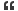# Thread: Missing number in the series

1. ## Missing number in the series

What is the missing number in the series?
4,9,20,43,90??Reply With Quote

2. ## Re: Missing number in the series

Ans: 185.

diff between 4 and 9 is 5 (4+1)
diff between 9 and 20 is 11 (9+2)
diff between 20 and 43 is 23 (20+3)
diff between 43 and 90 is 47(43+4)

-- JamesReply With Quote

3. ## Re: Missing number in the series

James your approach is prefect and the solution for this problem is 185 as you found out.Reply With Quote

4. ## Re: Missing number in the seriesOriginally Posted by scottWhat is the missing number in the series?
4,9,20,43,90??
Another approach

(4*2) + 1 = 9
(9*2) + 2 = 20
(20*2) + 3 = 43
(43*2) + 4 = 90
(90*2) + 5 = 185Reply With Quote

5. ## Re: Missing number in the series

yep

current No - (90 + 5)

Prev No - 90

so 90 + 95 = 185Reply With Quote

6. ## Missing number in the seriesOriginally Posted by scottWhat is the missing number in the series?
4,9,20,43,90??
the next no of the series is 143Reply With Quote

7. ## Missing number in the seriesOriginally Posted by scottWhat is the missing number in the series?
4,9,20,43,90??
the last no of the series is 143Reply With Quote

8. ##Re: Missing number in the series

4 9 20 43 90 ?

the difference

4 9 20 43 90 ?

5 11 23 47 ?

6 12 24 48

so the 3rd series is 6 12 24 (48) it doubles the digit.. adding 48 in 47 so the figure is 95 now we got main difference series.....adding 95 in main series 90 then ans is 185.Reply With Quote

9. ## Re: Missing number in the series

Hi,

The missing number is 185.

Here is the explanation

4 9 20 43 90

9-4=5
20-9=11
43-20=23
90-43=47

(5*2)+1=11
(11*2)+1=23
(23*2)+1=47

so, in the same way (47*2)+1=95

So, x-95=90.

Therefore x= 185.

ThanksReply With Quote

10. ##Re: Missing number in the seriesOriginally Posted by scottWhat is the missing number in the series?
4,9,20,43,90??Reply With Quote

11. ## Re: Missing number in the seriesReply With Quote

12. ## Re: Missing number in the seriesReply With Quote

13. ## Re: Missing number in the series

How can I draw up a formula that number series?

4,9,20,43,90??
Thank you!Reply With Quote

14. ## Re: Missing number in the series

Hi Isabele,

The formula is defined as
The difference between 9-4= 4+1 is 5
20-9=(5+5)+1 is 11
43-20=(11+11)+1 is 23
90-43=(23+23)+1 is 47
185-90=(47+47)+1 is 95Reply With Quote

15. ## Re: Missing number in the series

4 + 4 + 1 = 9
9 + 9 + 2 = 20
20 + 20 + 3 = 43
43 + 43 + 4 = 90
90 + 90 + 5 = 185Reply With Quote

16. ## Re: Missing number in the series

185
In this series each 2nd no is incremented by 1 with double of the previous number.
9=4*2+1
20=9*2+2
43=20*2+3
90=43*2+4
so, next number will be 185 as
185=90*2+5Reply With Quote

17.

####Posting Permissions

• You may not post new threads
• You may not post replies
• You may not post attachments
• You may not edit your posts
•